The SSM Procedure

Likelihood Computation and Model Fitting Phase

In view of the Gaussian nature of the response vector, the likelihood of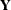can be computed by using the prediction-error decomposition. In the diffuse case the definition of the likelihood depends on the treatment of the diffuse quantities—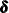,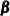, and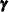. In the SSM procedure a likelihood called the diffuse-likelihood,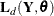, is used for parameter estimation. In the literature the diffuse likelihood is also called the restricted-likelihood. The diffuse likelihood is computed by treating the diffuse quantities as zero-mean, Gaussian, random variables with infinite variance (that is, they have diffuse distribution). In terms of the quantities described in Table 27.5 the diffuse likelihood is defined as follows: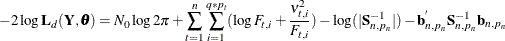where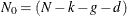,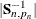denotes the determinant of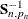, and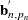denotes the transpose of the column vector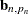. In the preceding formula, the terms that are associated with the missing response values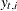are excluded and
denotes the total number of nonmissing response values in the sample. If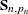is not invertible, then a generalized inverse is used in place of, andis computed based on the nonzero eigenvalues of. Moreover, in this case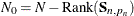. When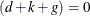, the terms that involveandare absent.

In addition to reporting the diffuse likelihood, the SSM procedure reports a variant of the likelihood called the profile likelihood. The profile likelihood is computed by treating the diffuse quantities—,, and—as unknown parameters (similar to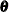). Interestingly, the quantities that are described in Table 27.5 play a key role in the computation of this variant of the likelihood also. It turns out that the filtering process yields the maximum likelihood (ML) estimates of,, andconditional on the remaining parameters of the model—. Moreover, the likelihood that is evaluated at the ML estimates of,, and—that is, the likelihood from which these parameters are profiled out—has the following expression: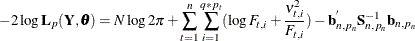Note that, computationally, the profile likelihood differs from the diffuse likelihood in only two respects: the constant term involves—the total number of nonmissing response values—rather than
, and the log-determinant term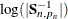is absent. However, in terms of theoretical considerations, the diffuse likelihood and the profile likelihood differ in an important way. It can be shown that the diffuse likelihood corresponds to the (nondiffuse) likelihood of a suitable transformation of. The transformation is chosen in such a way that the distribution of the transformed data no longer depends on the initial conditionand the regression vectorsand. In this sense, the diffuse likelihood is a pseudo-likelihood of the original data. The profile likelihood, on the other hand, does not involve any data transformation and can be considered as the likelihood of the original data. Of course, if the state space model fordoes not involve any diffuse quantities, then the two likelihoods are the same.

As noted earlier, the SSM procedure does not use the profile likelihood for parameter estimation. When the model specification contains any unknown parameters, they are estimated by maximizing the diffuse likelihood function. This is done by using a nonlinear optimization process that involves repeated evaluations ofat different values of. The maximum likelihood (ML) estimate ofis denoted by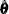. Because the diffuse likelihood is also called the restricted likelihood,is sometimes called the restricted maximum likelihood (REML) estimate. Approximate standard errors ofare computed by taking the square root of the diagonal elements of its (approximate) covariance matrix. This covariance is computed as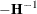, whereis the Hessian (the matrix of the second-order partials) of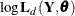evaluated at the optimum. It is known that the ML (or REML) estimate ofbased on the diffuse likelihood as well as the profile likelihood is consistent and efficient under mild regularity assumptions (as the number of distinct time points tend toward infinity). In addition, it is known that the estimate based on the diffuse likelihood is better in terms of having smaller bias.

Let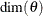denote the dimension of the parameter vector. After the parameter estimation is completed, PROC SSM prints the "Likelihood Computation Summary" table, which summarizes the likelihood calculations at, as shown in Table 27.6.

Table 27.6: Likelihood Computation Summary

Quantity

Formula

Nonmissing response values usedEstimated parametersInitialized diffuse state elements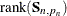Normalized residual sum of squares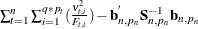Diffuse log likelihood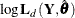Profile log likelihood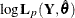In addition, the information criteria based on the diffuse likelihood and the profile likelihood are also reported. A variety of information criteria are reported. All these criteria are functions of twice the negative likelihood,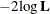(the likelihood can be either diffuse or profile);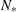, the effective sample size; and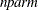, the effective number of model parameters. For the information criteria based on the diffuse likelihood, the effective sample size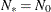and the effective number of model parameters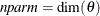. For the information criteria based on the profile likelihood, the effective sample size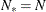and the effective number of model parameters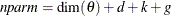. Table 27.7 summarizes the reported information criteria in smaller-is-better form.

Table 27.7: Information Criteria

Criterion

Formula

Reference

AIC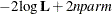Akaike (1974)

AICC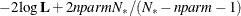Hurvich and Tsai (1989)

Burnham and Anderson (1998)

HQIC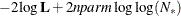Hannan and Quinn (1979)

BIC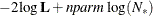Schwarz (1978)

CAIC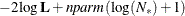Bozdogan (1987)